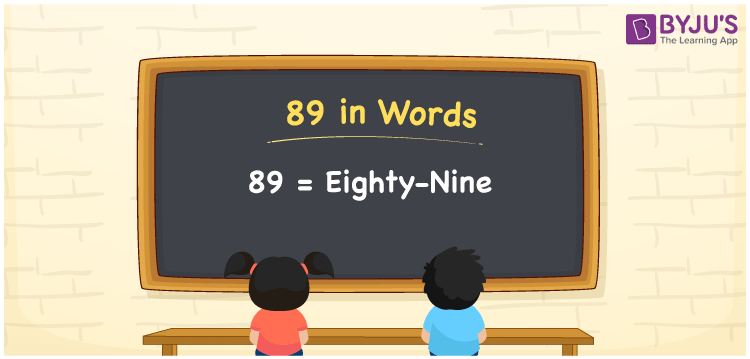# 89 in Words

89 in words is Eighty-Nine. The number name of 89 can be written using a place value chart. Hence, the place value chart helps us to represent the number 89 in words. We know that 89 is a cardinal number as it shows a certain amount. Learn the spelling of 89 in words using the English alphabet here.

 89 in words Eighty-Nine Eighty-Nine in numerical form 89

## 89 in English Words

Generally, we write numbers in words with the help of the English alphabet. Hence, 89 in words is written as Eighty-Nine.## How to Write 89 in Words?

Using the place value, we can convert 89 to words. Hence, it is essential to determine the place value of each digit of the given number. The place value chart for the number 89 is shown below.

 Tens Ones 8 9

Hence, we can write the expanded form as:

8 × Ten + 9 × One

= 8 x 10 + 9 x 1

= 80 + 9

= 89

= Eighty-Nine

Thus, 89 in words is written as Eighty-Nine.

Interesting way of writing 89 in words

8 = Eight

9 = Nine

80 = Eighty

80 + 9 = 89

Eighty + Nine = Eighty-Nine

Hence, the word form of the number 89 is Eighty-Nine.

89 is a natural number that is succeeded by 88 and preceded by 90

• 89 in words – Eighty-Nine
• Is 89 an odd number? – Yes
• Is 89 an even number? – No
• Is 89 a perfect square number? – No
• Is 89 a perfect cube number? – No
• Is 89 a prime number? – Yes
• Is 89 a composite number? – No

## Frequently Asked Questions on 89 in Words

Q1

### Write 89 in English.

89 in English can be written as Eighty-Nine.
Q2

### Find the value of 80 plus 9 and write the answer in words.

80 + 9 = 89 Hence, the value of 80 plus 9 in words can be written as Eighty-Nine.
Q3

### 89 is an odd number. True or False.

True, 89 is an odd number.